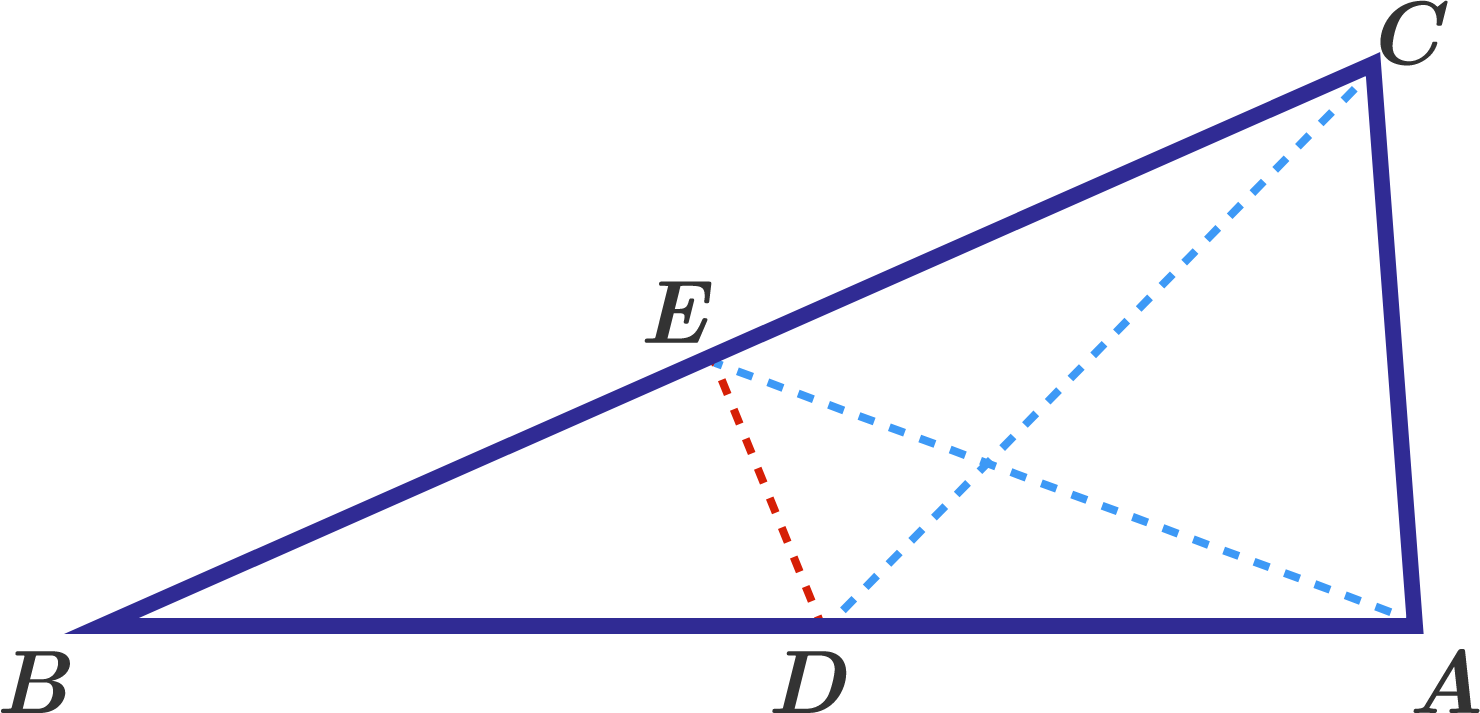Geometry Level 5In a triangle $ABC$ $\angle A =84^\circ,$ $\angle C=78^\circ.$ Points $D$ and $E$ are taken on the sides $AB$ and $BC,$ so that $\angle ACD =48^\circ,$ $\angle CAE =63^\circ.$ What is the measure (in degrees) of $\angle CDE$ ?

×